# 数据结构与算法之美笔记 : 跳表

Redis 中的有序集合（Sorted Set）就是用跳表来实现的.

## 如何理解“跳表”？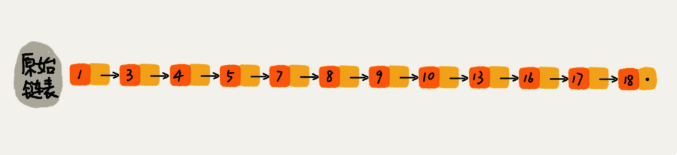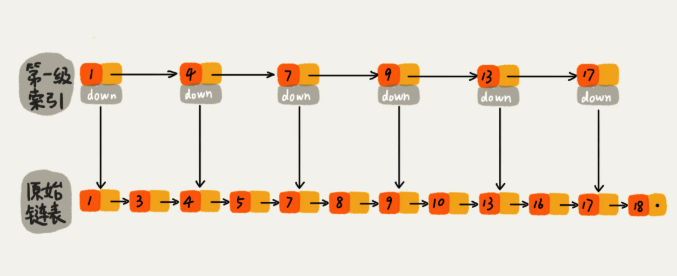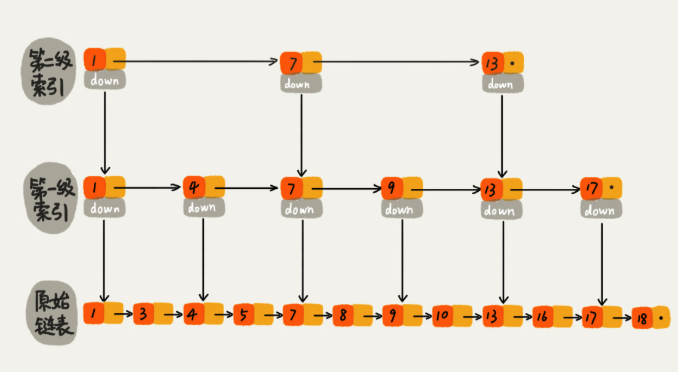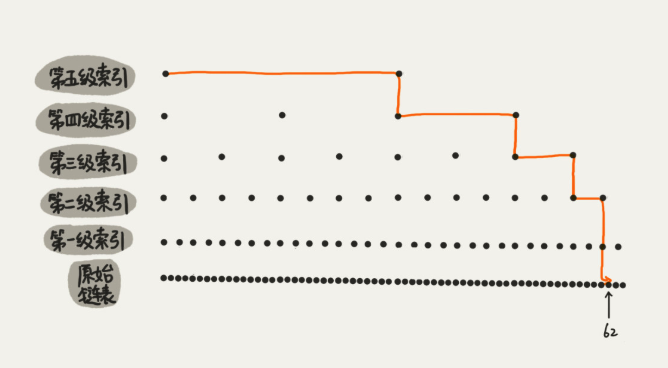## 用跳表查询到底有多快？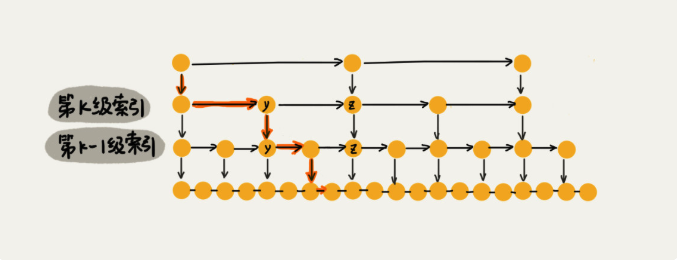## 跳表是不是很浪费内存？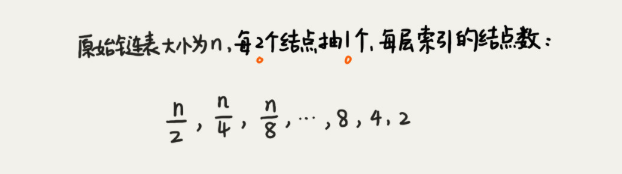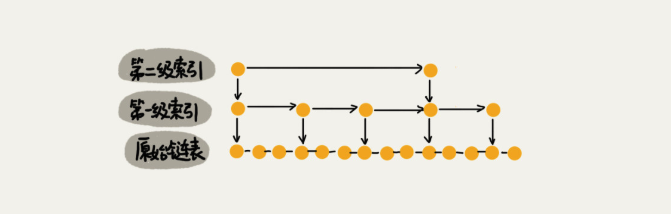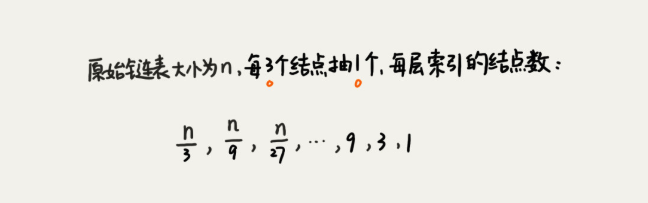## 高效的动态插入和删除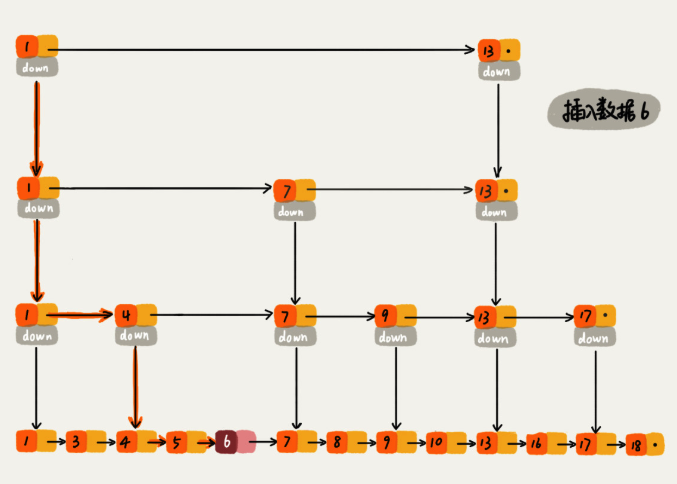## 跳表索引动态更新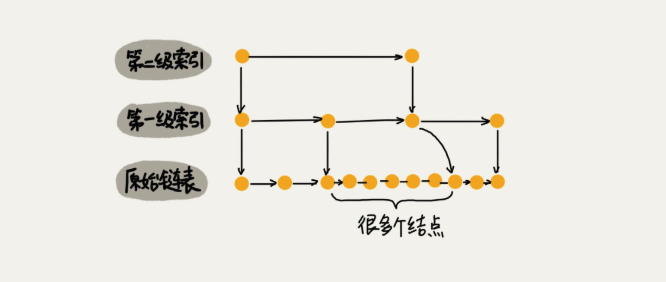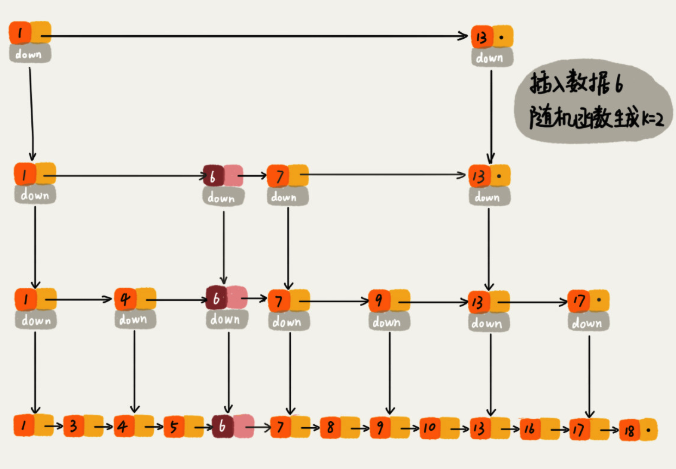package skiplist;

import java.util.Random;

/**
* 跳表的一种实现方法。
* 跳表中存储的是正整数，并且存储的是不重复的。
*
* Author：ZHENG
*/
public class SkipList {

private static final int MAX_LEVEL = 16;

private int levelCount = 1;

private Node head = new Node();  // 带头链表

private Random r = new Random();

public Node find(int value) {
for (int i = levelCount - 1; i >= 0; --i) {
while (p.forwards[i] != null && p.forwards[i].data < value) {
p = p.forwards[i];
}
}

if (p.forwards != null && p.forwards.data == value) {
return p.forwards;
} else {
return null;
}
}

public void insert(int value) {
int level = randomLevel();
Node newNode = new Node();
newNode.data = value;
newNode.maxLevel = level;
Node update[] = new Node[level];
for (int i = 0; i < level; ++i) {
}

// record every level largest value which smaller than insert value in update[]
for (int i = level - 1; i >= 0; --i) {
while (p.forwards[i] != null && p.forwards[i].data < value) {
p = p.forwards[i];
}
update[i] = p;// use update save node in search path
}

// in search path node next node become new node forwords(next)
for (int i = 0; i < level; ++i) {
newNode.forwards[i] = update[i].forwards[i];
update[i].forwards[i] = newNode;
}

// update node hight
if (levelCount < level) levelCount = level;
}

public void delete(int value) {
Node[] update = new Node[levelCount];
for (int i = levelCount - 1; i >= 0; --i) {
while (p.forwards[i] != null && p.forwards[i].data < value) {
p = p.forwards[i];
}
update[i] = p;
}

if (p.forwards != null && p.forwards.data == value) {
for (int i = levelCount - 1; i >= 0; --i) {
if (update[i].forwards[i] != null && update[i].forwards[i].data == value) {
update[i].forwards[i] = update[i].forwards[i].forwards[i];
}
}
}
}

// 随机 level 次，如果是奇数层数 +1，防止伪随机
private int randomLevel() {
int level = 1;
for (int i = 1; i < MAX_LEVEL; ++i) {
if (r.nextInt() % 2 == 1) {
level++;
}
}

return level;
}

public void printAll() {
while (p.forwards != null) {
System.out.print(p.forwards + " ");
p = p.forwards;
}
System.out.println();
}

public class Node {
private int data = -1;
private Node forwards[] = new Node[MAX_LEVEL];
private int maxLevel = 0;

@Override
public String toString() {
StringBuilder builder = new StringBuilder();
builder.append("{ data: ");
builder.append(data);
builder.append("; levels: ");
builder.append(maxLevel);
builder.append(" }");

return builder.toString();
}
}

}


Redis 中的有序集合是通过跳表来实现的，严格点讲，其实还用到了散列表。不过散列表我们后面才会讲到，所以我们现在暂且忽略这部分。如果你去查看 Redis 的开发手册，就会发现，Redis 中的有序集合支持的核心操作主要有下面这几个：

• 插入一个数据；

• 删除一个数据；

• 查找一个数据；

• 按照区间查找数据（比如查找值在 [100, 356] 之间的数据）；

• 迭代输出有序序列。

## 内容小结©️2019 CSDN 皮肤主题: 精致技术 设计师: CSDN官方博客